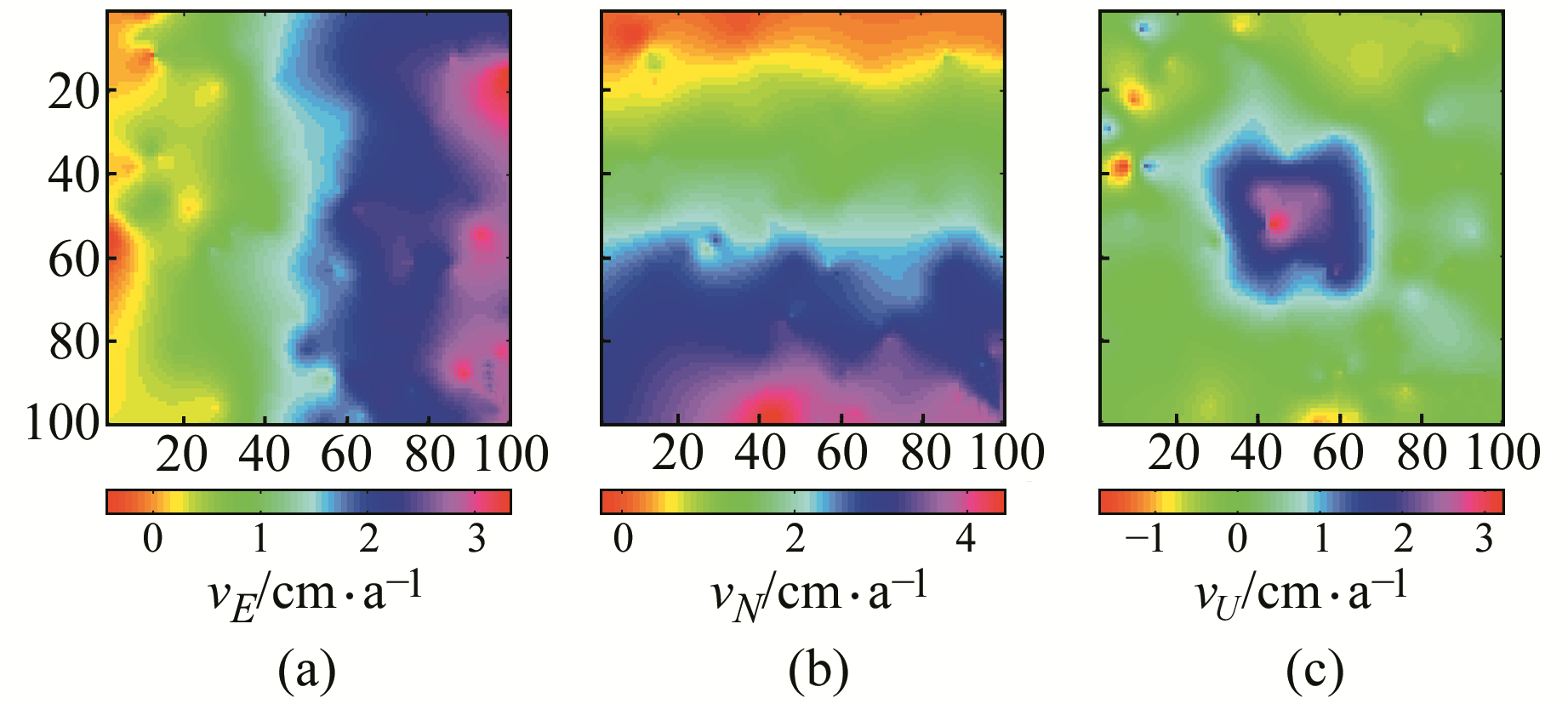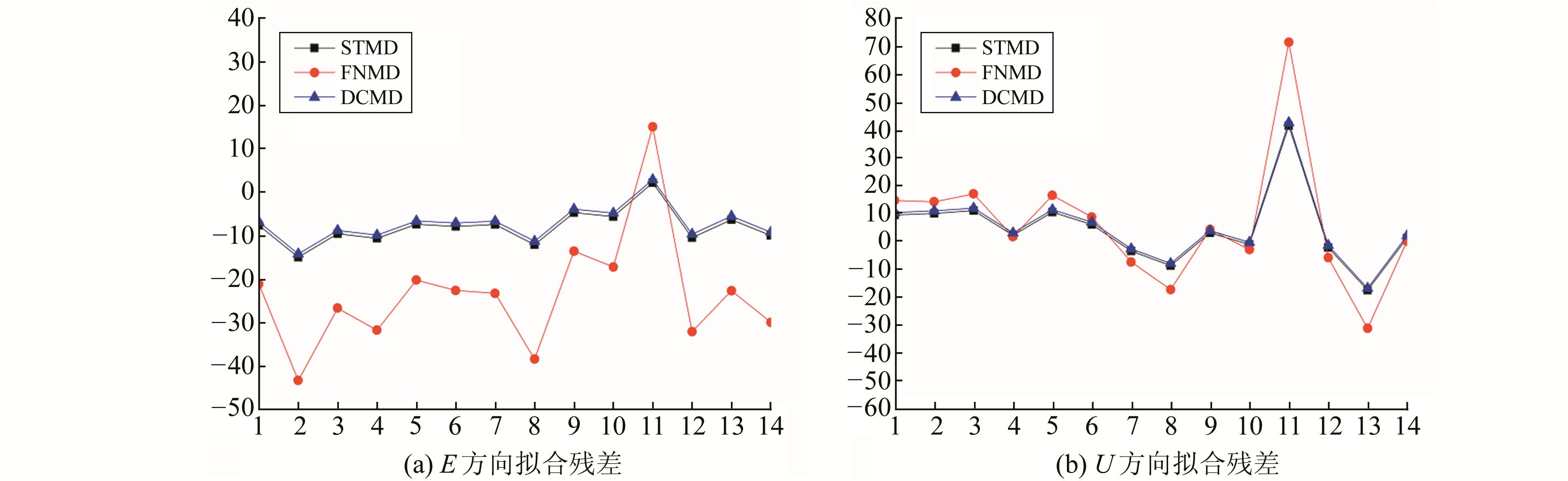﻿ 函数模型与随机模型双约束的GPS-InSAR数据融合方法建立三维形变场文章快速检索 高级检索
 大地测量与地球动力学2019, Vol. 39Issue (11): 1112-1117  DOI: 10.14075/j.jgg.2019.11.003### 引用本文MA Yange, ZHAO Lihua, ZHANG Panpan, et al. Establishment of 3D Deformation Field by GPS-InSAR Data Fusion Method Based on Function and Stochastic Model[J]. Journal of Geodesy and Geodynamics, 2019, 39(11): 1112-1117.### Foundation support

National Natural Science Foundation of China, No. 41604001, 41674001, 41504005, 41574003;Special Fund for Basic Scientific Research of Central Universities, No. CHD300102268204, 310826172202.

### Corresponding author

ZHAO Lihua, PhD, associate professor, majors in geodesy, surveying and measurement data processing, E-mail:zhaolih@chd.edu.cn.

### 第一作者简介

MA Yange, postgraduate, majors in GPS-InSAR data processing, E-mail: mygzpp@163.com.

### 文章历史

1. 长安大学地质工程与测绘学院，西安市雁塔路126号，710054

InSAR作为当前重要的空间对地形变观测手段，能够获取高精度区域地表形变及大范围地壳运动特征等信息，较常规的地壳形变监测方法能更清晰地反映观测区的整体形变情况。但因存在视线(LOS)向模糊的问题，InSAR只能获取雷达脉冲入射方向的一维地表形变量，无法满足地表三维形变的定量描述。GPS技术具有高时间分辨率、高精度的地表三维监测优势，近年来利用InSAR-GPS技术融合解算三维形变场一直是InSAR技术监测地表形变研究的热点。已有学者在不同对地观测手段融合监测三维形变场的方面进行了研究[1-3]，为InSAR和GPS两种类型的数据融合提供了很好的思路。

1 函数模型与随机模型双约束的GPS-InSAR融合模型

InSAR在某一点P处的LOS向形变观测量为LInSAR，根据雷达成像的几何关系建立观测方程:

 ${\mathit{\boldsymbol{V}}_{{\rm{InSAR}}}} = {\mathit{\boldsymbol{A}}_{{\rm{InSAR}}}}\mathit{\boldsymbol{\hat X}} - {\mathit{\boldsymbol{L}}_{{\rm{InSAR}}}}$ (1)

 $\left\{ \begin{array}{l} {\mathit{\boldsymbol{V}}_{\mathit{\boldsymbol{\bar X}}}} = \mathit{\boldsymbol{\hat X}} - \mathit{\boldsymbol{\bar X}}\\ {\mathit{\boldsymbol{V}}_{{\rm{InSAR}}}} = {\mathit{\boldsymbol{A}}_{{\rm{InSAR}}}}\mathit{\boldsymbol{\hat X}} - {\mathit{\boldsymbol{L}}_{{\rm{InSAR}}}} \end{array} \right.$ (2)

 $\mathit{\boldsymbol{\hat X}} = \mathit{\boldsymbol{N}}_{bb}^{ - 1}\mathit{\boldsymbol{G}}$ (3)

$\mathit{\boldsymbol{\widehat X}}$的协因数矩阵为：

 ${\mathit{\boldsymbol{Q}}_{{{\hat X}_S}}} = \mathit{\boldsymbol{N}}_{bb}^{ - 1}$ (4)

 $\left\{ {\begin{array}{*{20}{l}} {{\mathit{\boldsymbol{V}}_{{\rm{InSAR}}}} = {\mathit{\boldsymbol{A}}_{{\rm{InSAR}}}}\mathit{\boldsymbol{\hat X}} - {\mathit{\boldsymbol{L}}_{{\rm{InSAR}}}}}\\ {\mathit{\boldsymbol{C\hat X}} - \mathit{\boldsymbol{W}} = 0} \end{array}} \right.$ (5)

 $\begin{array}{l} \mathit{\boldsymbol{\hat X}} = \left( {\mathit{\boldsymbol{N}}_b^{ - 1} - \mathit{\boldsymbol{N}}_b^{ - 1}{\mathit{\boldsymbol{C}}^{\rm{T}}}\mathit{\boldsymbol{N}}_c^{ - 1}\mathit{\boldsymbol{CN}}_b^{ - 1}} \right) \cdot \\ \left( {\mathit{\boldsymbol{A}}_{{\rm{InSAR}}}^{\rm{T}}{\mathit{\boldsymbol{P}}_{{\rm{InSAR}}}}{\mathit{\boldsymbol{L}}_{{\rm{InSAR}}}}} \right) - \mathit{\boldsymbol{N}}_b^{ - 1}{\mathit{\boldsymbol{C}}^{\rm{T}}}\mathit{\boldsymbol{N}}_c^{ - 1}\mathit{\boldsymbol{W}} \end{array}$ (6)
 ${\mathit{\boldsymbol{K}}_S} = \mathit{\boldsymbol{N}}_c^{ - 1}\left( {\mathit{\boldsymbol{CN}}_b^{ - 1}\mathit{\boldsymbol{A}}_{{\rm{InSAR}}}^{\rm{T}}{\mathit{\boldsymbol{P}}_{{\rm{InSAR}}}}{\mathit{\boldsymbol{L}}_{{\rm{InSAR}}}} + \mathit{\boldsymbol{W}}} \right)$ (7)

$\mathit{\boldsymbol{\widehat X}}$的协因数矩阵为：

 ${\mathit{\boldsymbol{Q}}_{{{\hat X}_F}}} = \mathit{\boldsymbol{N}}_b^{ - 1} - \mathit{\boldsymbol{N}}_b^{ - 1}{\mathit{\boldsymbol{C}}^T}\mathit{\boldsymbol{N}}_c^{ - 1}\mathit{\boldsymbol{CN}}_b^{ - 1}$ (8)

 $\left\{ {\begin{array}{*{20}{l}} {{\mathit{\boldsymbol{V}}_{{\rm{InSAR}}}} = {\mathit{\boldsymbol{A}}_{{\rm{InSAR}}}}\mathit{\boldsymbol{\hat X}} - {\mathit{\boldsymbol{L}}_{{\rm{InSAR}}}}}\\ {{\mathit{\boldsymbol{V}}_{\bar X}} = \mathit{\boldsymbol{\hat X}} - \mathit{\boldsymbol{\bar X}}}\\ {\mathit{\boldsymbol{C\hat X}} - \mathit{\boldsymbol{W}} = 0} \end{array}} \right.$ (9)

 $\begin{array}{*{20}{l}} {\mathit{\boldsymbol{\hat X}} = \mathit{\boldsymbol{N}}_{bb}^{ - 1}\left( {\mathit{\boldsymbol{G}} - {\mathit{\boldsymbol{C}}^{\rm{T}}}{\mathit{\boldsymbol{K}}_S}} \right) = }\\ {\mathit{\boldsymbol{N}}_{bb}^{ - 1}\mathit{\boldsymbol{G}} - \mathit{\boldsymbol{N}}_{bb}^{ - 1}{\mathit{\boldsymbol{C}}^{\rm{T}}}{\mathit{\boldsymbol{K}}_S} = {{\mathit{\boldsymbol{\hat X}}}_D}} \end{array}$ (10)
 ${\mathit{\boldsymbol{K}}_S} = \mathit{\boldsymbol{N}}_\alpha ^{ - 1}\left( {\mathit{\boldsymbol{CN}}_{bb}^{ - 1}\mathit{\boldsymbol{G}} - \mathit{\boldsymbol{W}}} \right)$ (11)

$\mathit{\boldsymbol{\widehat X}}$的协因数矩阵为：

 ${\mathit{\boldsymbol{Q}}_{{{\hat X}_D}}} = \mathit{\boldsymbol{N}}_{bb}^{ - 1} - \mathit{\boldsymbol{N}}_{bb}^{ - 1}{\mathit{\boldsymbol{C}}^{\rm{T}}}{\left( {\mathit{\boldsymbol{CN}}_{cc}^{ - 1}{\mathit{\boldsymbol{C}}^{\rm{T}}}} \right)^{ - 1}}\mathit{\boldsymbol{CN}}_{bb}^{ - 1}$ (12)

 ${\mathit{\boldsymbol{Q}}_{{{\hat X}_D}}} = {\mathit{\boldsymbol{Q}}_{{{\hat X}_S}}} - \mathit{\boldsymbol{N}}_{bb}^{ - 1}{\mathit{\boldsymbol{C}}^{\rm{T}}}{\left( {\mathit{\boldsymbol{CN}}_{bb}^{ - 1}{\mathit{\boldsymbol{C}}^{\rm{T}}}} \right)^{ - 1}}\mathit{\boldsymbol{CN}}_{bb}^{ - 1}$ (13)

 ${\rm{tr}}\left( {{\mathit{\boldsymbol{Q}}_{{{\hat X}_D}}}} \right) \le {\rm{tr}}\left( {{\mathit{\boldsymbol{Q}}_{{{\hat X}_S}}}} \right)$ (14)

 ${\rm{tr}}\left( {{\mathit{\boldsymbol{Q}}_{{{\hat X}_D}}}} \right) \le {\rm{tr}}\left( {{\mathit{\boldsymbol{Q}}_{{{\hat X}_F}}}} \right)$ (15)

2 算例分析 2.1 模拟数据进行算例分析

 $\left[ {\begin{array}{*{20}{c}} {{V_x}}\\ {{V_y}}\\ {{V_z}} \end{array}} \right] = \left[ {\begin{array}{*{20}{c}} {V_x^0x}\\ {V_y^0y}\\ {\left[ {a + 2{\rm{ \mathit{ π} }}b\cos \left( {2{\rm{ \mathit{ π} }}t} \right)} \right]{{\rm{e}}^{ - \frac{{{x^2} + {y^2}}}{w}}}} \end{array}} \right]$ (16)图 1 模拟的地表三维形变 Fig. 1 Simulated 3D surface deformation图 2 模拟的加噪后InSAR监测的地表形变 Fig. 2 Ground deformation monitored by InSAR after simulated noise图 3 克里金插值法得到的三维形变场 Fig. 3 Three dimensional deformation field obtained by Kriging interpolation

1) 方案1，以GPS的先验观测值为参数的随机模型条件，以InSAR的LOS向形变值为观测量，进行随机模型约束的GPS与InSAR数据融合(STMD)。

2) 方案2，以三维形变的南北向观测值作为条件约束，进行函数模型约束的GPS与InSAR数据融合(FNMD)。

3) 方案3，以GPS的先验观测值为参数的随机模型条件，同时以三维形变的南北向观测值作为条件约束，进行函数模型与随机模型双约束的GPS与InSAR数据融合(DCMD)。表 1 不同计算方法在ENU方向的均方根误差 Tab. 1 Root mean square error of E, N and U directions in different calculation methods
2.2 实测数据算例分析图 4 InSAR数据和GPS点位分布 Fig. 4 InSAR data and GPS point distribution表 2 不同方案ENU方向拟合精度 Tab. 2 Fitting accuracy of E, N and U in different schemes图 5 E和U方向拟合残差 Fig. 5 E and U direction fitting residuals表 3 不同方案计算参数协因数的迹 Tab. 3 Traces of different schemes cofactors表 4 ENU方向计算形变速率与实测形变速率比较结果统计 Tab. 4 Comparison of calculated deformation rate in ENU direction with measured deformation rate results statistics图 6 三维形变场 Fig. 6 Three dimensional deformation field

1) 直接分解法在EN方向的精度与克里金插值法相同，在U方向的精度则优于克里金插值法。

2) 在EU方向，双模型约束与单一的随机模型约束的精度优于常规的直接分解法，其中U方向的精度提高最为显著。

3) 函数模型约束在E方向相较于其他几种方法精度较低，主要原因是在利用N方向进行强制约束时，EU方向的形变信息主要来源于InSAR数据源，故平面精度较低。U方向精度相较于克里金插值法提升较多，进一步说明InSAR对于高程信息较为敏感。E方向精度较差，说明对于东西方向的形变不能单靠InSAR信息获得，而是要充分地利用GPS数据。

4) 双模型约束的精度优于单一函数模型约束和单一随机模型约束的精度，即双模型约束的精度优于单一模型约束的精度。但双模型约束较单一模型约束的精度提高有限，因为研究区GPS点数量非常有限且分布不均匀，对GPS的内插精度影响很大，且没有考虑InSAR观测数据中系统误差的影响。

5) 随机模型约束和双模型约束计算的三维形变场运动趋势基本保持一致，而函数模型约束与随机模型约束、双模型约束在EU方向存在明显差异。研究区有着向东南方向运动的趋势，与渭河盆地向东南方向运动的结论一致。从图 6(c)6(h)可以看出，研究区的西南方向主要发生沉降。

3 结语

  Samsonov S V, Tiampo K F, Rundle J B, et al. Application of DInSAR-GPS Optimization for Derivation of Fine-Scale Surface Motion Maps of Southern California[J]. IEEE Transactions on Geoscience and Remote Sensing, 2007, 45(2): 512-521 DOI:10.1109/TGRS.2006.887166 (0)  罗海滨, 何秀凤. GPS-DInSAR集成监测的改进定权方法与仿真实验分析[J]. 煤炭学报, 2012, 37(10): 1 612-1 617 (Luo Haibin, He Xiufeng. Improved Determining Weight Method for GPS-DInSAR Integration Monitoring and Simulation Experiment Analysis[J]. Journal of China Coal Society, 2012, 37(10): 1 612-1 617) (0)  曹海坤, 赵丽华, 毕研磊. 利用附加系统参数的GPS-InSAR综合形变模型建立三维形变场[J]. 大地测量与地球动力学, 2017, 37(4): 344-348 (Cao Haikun, Zhao Lihua, Bi Yanlei. GPS-InSAR Integrated Deformation Model with Additional Systematic Parameters for Three-Dimensional Deformation Fields[J]. Journal of Geodesy and Geodynamics, 2017, 37(4): 344-348) (0)  Samsonov S V, Tiampo K F, Rundle J B. Application of DInSAR-GPS Optimization for Derivation of Three-Dimensional Surface Motion of the Southern California Region along the San Andreas Fault[J]. Computers and Geosciences, 2008, 34(5): 503-514 DOI:10.1016/j.cageo.2007.05.013 (0)  Guglielmino F, Nunnari G, Puglisi G, et al. Simultaneous and Integrated Strain Tensor Estimation from Geodetic and Satellite Deformation Measurements to Obtain Three-Dimensional Displacement Maps[J]. IEEE Transactions on Geoscience and Remote Sensing, 2011, 49(6): 1 815-1 826 DOI:10.1109/TGRS.2010.2103078 (0)  Guglielmino F, Bignami C, Bonforte A, et al. Analysis of Satellite and in Situ Ground Deformation Data Integrated by the SISTEM Approach: The April 3, 2010 Earthquake Along the Pernicana Fault(Mt. Etna-Italy) Case Study[J]. Earth and Planetary Science Letters, 2011, 312(3-4): 327-336 DOI:10.1016/j.epsl.2011.10.028 (0)  张永志. 位错理论及其在大地变形研究中的应用[M]. 西安: 西安交通大学出版社, 2011 (Zhang Yongzhi. Dislocation Theory and Its Application in the Study of Geodetic Deformation[M]. Xi'an: Xi'an Jiaotong University Press, 2011) (0)  刘斌, 张景发, 罗毅, 等. 基于SAR影像构建三维同震形变场方法研究[J]. 大地测量与地球动力学, 2013, 33(4): 4-8 (Liu Bin, Zhang Jingfa, Luo Yi, et al. Study Reconstructing of 3D Coseismic Deformation Field Based on SAR Image[J]. Journal of Geodesy and Geodynamics, 2013, 33(4): 4-8) (0)
Establishment of 3D Deformation Field by GPS-InSAR Data Fusion Method Based on Function and Stochastic Model
MA Yange1ZHAO Lihua1ZHANG Panpan1     LV Jianing1
1. School of Geology Engineering and Geomatics, Chang'an University, 126 Yanta Road, Xi'an 710054, China
Abstract: Aiming at the problem of insufficient monitoring information when InSAR technology is used to study the surface three-dimensional deformation, a three-dimensional deformation model with stochastic model constraints is established with GPS monitoring information as a priori. Considering that the LOS observation is insensitive to the North-South deformation due to the polar orbit of SAR satellite, the function constraint condition of 3D deformation solution is constructed with the strong constraint of GPS north-south deformation observation. The results of the simulation data and the measured data in Xi'an area show that the least squares solution based on the combination of stochastic model and functional model is more accurate than the parametric solution, with only functional model constraint or stochastic model constraint, and the parametric solution without any constraint.
Key words: InSAR; GPS; stochastic model; function model; double constraint model; three-dimensional deformation